##### The Japanese Maple specialist
Direct order Contact Help / Services Newsletter# Shelf to displaySearch

Japanese MaplesBonsai soil and fertiliserAcer seeds and more Young maples and bonsaiHostasWind bells and chimesSaxifragesVarieties introduced into Europe

Packing and shipping charges

# Maimori

› Japanese maples › Acer palmatum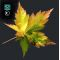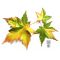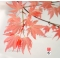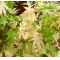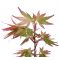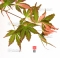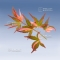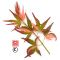ref. : 631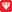Choose the right size
Pot size

This item is temporarily unavailable

###### Description

Brown spring foliage then beginning May he/it becomes multicolored of orange, green rose, cream on some leaves. In been plume of green clear, red in fall.Exceptional coloration indeed the variegation is always new of part the position of the colors on the leaf. We counsel you in order to keep the most beautiful variegations to fold back the non multicolored branches that are developed themselves.

As far as possible one will privilege the variegation rather than the height of the topic.

For a lasting variegation he/it is indispensable to supervise the growth of non multicolored shoots and to suppress them.

The delivered plant will have branched out little.

#multicolored 4.2 #variegation 4.1 #palmatum 2.8 #maimori 2.7 #green 2.5 #acer 2.4 #will 2.4 #indispensable 2.3 #variegations 2.2 #exceptional 2.1

###### Technical description
 Species Acer palmatum same species items Variety Maimori Leaf colour Variegated leaves same colour items Leaf shape Made tense same shaped items Adult height Between 2 and 4 meters same height items Port Fastigiate same port items Expositions Partial shade same exposition items

Formule
(( ROUND((CHAR_LENGTH(b.article_nom)-CHAR_LENGTH(REPLACE(b.article_nom, 'multicolored', '')))/LENGTH('multicolored')) + ROUND((CHAR_LENGTH(b.article_description)-CHAR_LENGTH(REPLACE(b.article_description, 'multicolored', '')))/LENGTH('multicolored')) ) * 4.2) + (( ROUND((CHAR_LENGTH(b.article_nom)-CHAR_LENGTH(REPLACE(b.article_nom, 'variegation', '')))/LENGTH('variegation')) + ROUND((CHAR_LENGTH(b.article_description)-CHAR_LENGTH(REPLACE(b.article_description, 'variegation', '')))/LENGTH('variegation')) ) * 4.1) + (( ROUND((CHAR_LENGTH(b.article_nom)-CHAR_LENGTH(REPLACE(b.article_nom, 'maimori', '')))/LENGTH('maimori')) + ROUND((CHAR_LENGTH(b.article_description)-CHAR_LENGTH(REPLACE(b.article_description, 'maimori', '')))/LENGTH('maimori')) ) * 2.7) + (( ROUND((CHAR_LENGTH(b.article_nom)-CHAR_LENGTH(REPLACE(b.article_nom, 'green', '')))/LENGTH('green')) + ROUND((CHAR_LENGTH(b.article_description)-CHAR_LENGTH(REPLACE(b.article_description, 'green', '')))/LENGTH('green')) ) * 2.5) + (( ROUND((CHAR_LENGTH(b.article_nom)-CHAR_LENGTH(REPLACE(b.article_nom, 'will', '')))/LENGTH('will')) + ROUND((CHAR_LENGTH(b.article_description)-CHAR_LENGTH(REPLACE(b.article_description, 'will', '')))/LENGTH('will')) ) * 2.4) + (( ROUND((CHAR_LENGTH(b.article_nom)-CHAR_LENGTH(REPLACE(b.article_nom, 'indispensable', '')))/LENGTH('indispensable')) + ROUND((CHAR_LENGTH(b.article_description)-CHAR_LENGTH(REPLACE(b.article_description, 'indispensable', '')))/LENGTH('indispensable')) ) * 2.3) + (( ROUND((CHAR_LENGTH(b.article_nom)-CHAR_LENGTH(REPLACE(b.article_nom, 'variegations', '')))/LENGTH('variegations')) + ROUND((CHAR_LENGTH(b.article_description)-CHAR_LENGTH(REPLACE(b.article_description, 'variegations', '')))/LENGTH('variegations')) ) * 2.2) + (( ROUND((CHAR_LENGTH(b.article_nom)-CHAR_LENGTH(REPLACE(b.article_nom, 'exceptional', '')))/LENGTH('exceptional')) + ROUND((CHAR_LENGTH(b.article_description)-CHAR_LENGTH(REPLACE(b.article_description, 'exceptional', '')))/LENGTH('exceptional')) ) * 2.1) + (( ROUND((CHAR_LENGTH(b.article_nom)-CHAR_LENGTH(REPLACE(b.article_nom, 'themselves', '')))/LENGTH('themselves')) + ROUND((CHAR_LENGTH(b.article_description)-CHAR_LENGTH(REPLACE(b.article_description, 'themselves', '')))/LENGTH('themselves')) ) * 2) + (( ROUND((CHAR_LENGTH(b.article_nom)-CHAR_LENGTH(REPLACE(b.article_nom, 'coloration', '')))/LENGTH('coloration')) + ROUND((CHAR_LENGTH(b.article_description)-CHAR_LENGTH(REPLACE(b.article_description, 'coloration', '')))/LENGTH('coloration')) ) * 2)

## Secure payment## Delivery

Our logistic partners :